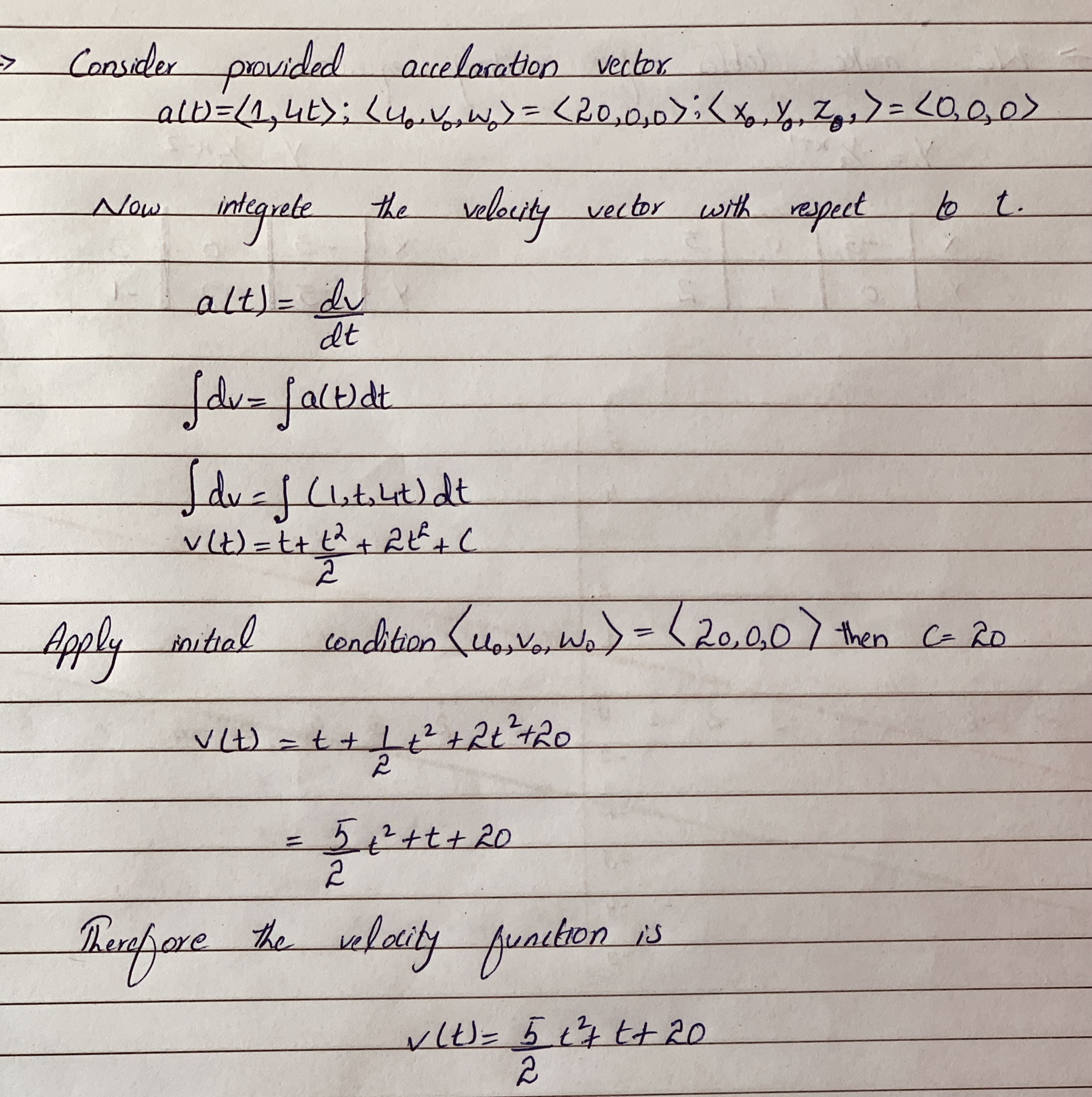sagnuhh

2021-01-02

Solving equations of motion Given an acceleration vector, initial velocity $⟨{u}_{0},{v}_{0},{w}_{0}⟩$, and initial position $⟨{x}_{0},{y}_{0},{z}_{0}⟩$, find the velocity and position vectors, for $t\ge 0$.
$a\left(t\right)=⟨1,t,4t⟩,⟨{u}_{0},{v}_{0},{w}_{0}⟩=⟨20,0,0⟩,⟨{x}_{0},{y}_{0},{z}_{0}⟩=⟨0,0,0⟩$okomgcae

...............................................